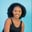Related Tags

dart
import
communitycreator

# How to use the import keyword in DartMaria Elijah

### Overview

In Dart, we use the import keyword to import libraries or other files within a program.

### Syntax

import library_name;


Assume we need some mathematical constant such as pi or a function like sqrt() in our program, then we have to import the dart: math.

### Code

The following code shows how to use the import keyword in Dart.

// import keyword in dart
import 'dart:math';
void main() {
var cir = 2 *pi * radius;
print("The circumference of a circle: $cir"); // using sqrt() to find square root of 81 print("The square root of 81 is:${sqrt(81)}");
}

### Explanation

• Line 2: We import the dart: math library to access the pi and the sqrt() function.
• Line 3: We create a main() function.
• Line 4: We declare a variable named radius and assign a value to it.
• Line 5: We declare a variable named cir which holds the value from the computation.
• Line 6: We display the result using print().
• Line 8: We calculate the square root of 81 using the sqrt() function from the dart:math library. Finally, we display the result.

RELATED TAGS

dart
import
communitycreator

CONTRIBUTORMaria Elijah
RELATED COURSES

View all Courses

Keep Exploring

Learn in-demand tech skills in half the time